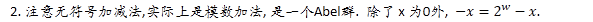# 溢出判断

(虽然本篇的代码经过大量的测试, 但本人仍然无法保证代码的正确性, 希望大家纠错).

[定理][理解]

;复制代码

/ Determine whether arguments can be added without overflow /
int uadd_ok(unsigned int x, unsigned int y)
{
return !(x+y < x);
}

[定理][理解]
1. 计算机中没有减法, x-y = x+(-y), 这里的-y就是上述的y的加法逆元. 不管是有符号还是无符号, 都是转换为加法运算. 只是加法逆元的定义不同.3. C语言保证 -x = ~x+1; 可以验证这种方式与上面公式等价.

1. s=x-y = x+(-y). 那么 不会溢出 等价于 y不为0 或者 !uadd_ok(x, -y)./ Determine whether argumnts can be substracted without overflow /
int usub_ok(unsigned int x, unsigned int y)
{
}

[定理][理解]

;复制代码

/ Determine whether arguments can be multiplied without overflow /
int umul_ok(unsigned int x, unsigned int y)
{
unsigned int p = x * y;
return !x || p/x==y;
}

[定理]

[理解]

/ Determine whether arguments can be added without overflow /
{
return !(x

[定理][理解]

;复制代码

/ Determine whether arguments can be subtracted without overflow /
int tsub_ok(int x, int y)
{

# if 0

if (y == INT_MIN)
return x

[定理]

/ Determine whether arguments can be multiplied without overflow. /
int tmul_ok(int x, int y)
{

# if 0

int p = x * y;
return !x || p/x==y;

# endif

return umul_ok(x, y); / 直接调用 /
}

Original: https://www.cnblogs.com/qiumingcheng/p/15915642.html
Author: 邱明成
Title: 溢出判断